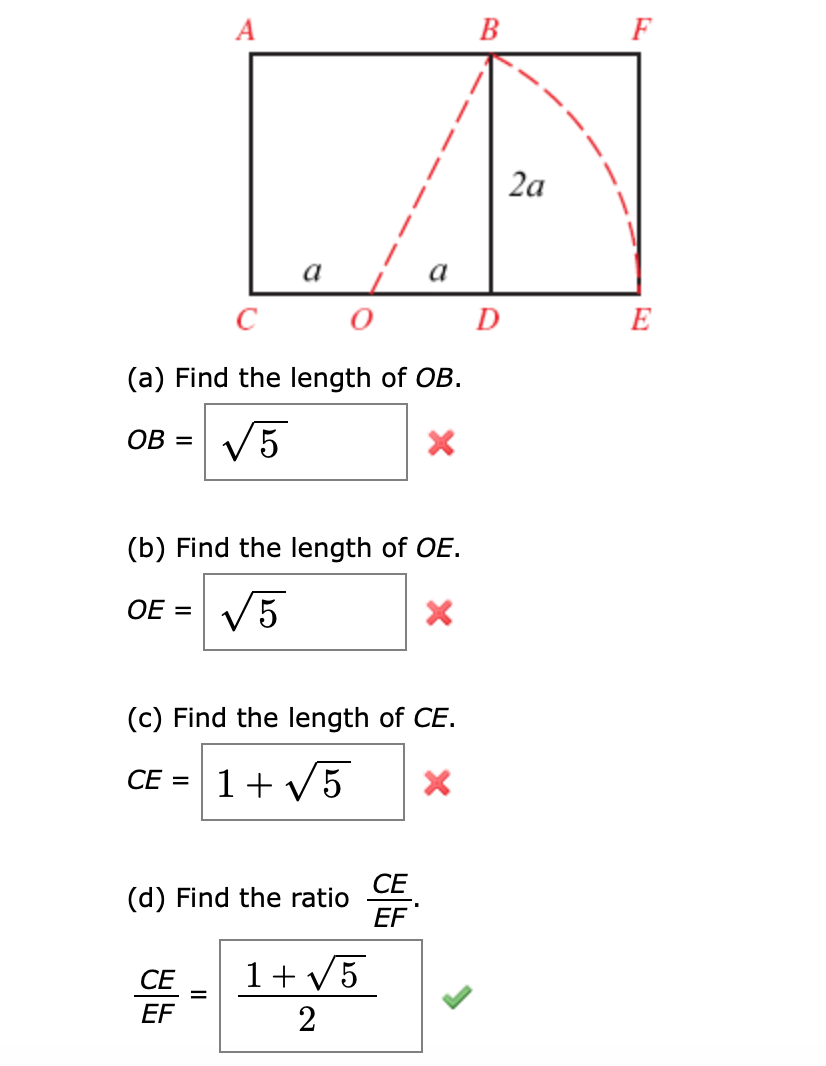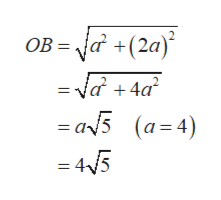FBA2aaaECOD(a) Find the length of OBV5OB =(b) Find the length of OEV5OE =(c) Find the length of CE.1 5CE =X(d) Find the ratio CEEF1CEEF2

Question

Rectangle ACEF shown in the figure is a golden rectangle. It is constructed from square ACDB by holding line segment OB fixed at point O and then letting point B drop down until OB aligns with CD. The ratio of the length to the width in the golden rectangle is called the golden ratio. Find the lengths below to arrive at the golden ratio. (Let a = 4.)help_outlineImage TranscriptioncloseF B A 2a a a E C O D (a) Find the length of OB V5 OB = (b) Find the length of OE V5 OE = (c) Find the length of CE. 1 5 CE = X (d) Find the ratio CE EF 1 CE EF 2 fullscreen
Step 1

According to given figure :

The l...help_outlineImage TranscriptioncloseOB a +(2a = va +4a = a5 (a=4) 4/5 fullscreen

Want to see the full answer?

See Solution

Want to see this answer and more?

Our solutions are written by experts, many with advanced degrees, and available 24/7

See Solution
Tagged in

Trigonometric Ratios